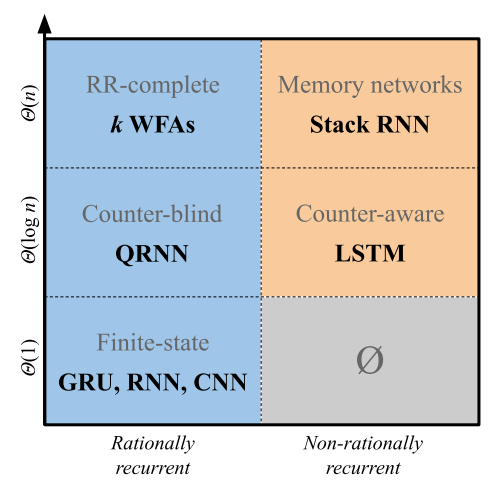Before the Transformer architecture was invented, recurrent networks were the most prominent architectures used in machine translation and the rest of natural language processing. It is quite surprising how little we still know about the architectures from the theoretical perspective. People often repeat a claim that recurrent networks are Turing complete, and therefore they can, in theory, perform any computation. This is so theoretical that it is not really true. As Yoav Goldberg explained in his talk in 2018 at EMNLP, such that even I understood it, the proof is based on the assumption that neural networks can use decimal places of real numbers for writing potentially infinite codes. The problem is the real real numbers in our digital computers can never have infinite precision, so the proof does not really say anything about the models we train.

LSTM networks invented in 1997 are probably the most frequently used recurrent architectures. Since 2014, they have a strong competitor, GRUs, that promise to be as good as LSTMs, but a little bit simpler. After all, they use the same trick to tackle the vanishing gradient problem with less computation. Empirical evaluations showed it did not really matter which of you choose.

A recent pre-print with title A Formal Hierarchy of RNN Architectures by authors from several institutions, but mostly Allen Institute for AI, introduces a formal hierarchy for the expressiveness of recurrent networks that sheds more light on how the architectures really differ. The hierarchy can be best described using Figure 1 from the paper:The paper introduces two concepts that describe the expressiveness of the recurrent networks: rationality and space complexity. It actually appears that there is a big theoretical difference between LSTMs and GRUs.

Rationality: Rational RNNs correspond to weighted finite state machines. You can imagine the machine as follows: The machine is in a state, it gets some input, and based on the input it has various options on how to change its inner state with different weights. The paper shows that vanilla RNNs and GRUs rational whereas LSTMs are stronger than that, i.e., it can perform computations that too complex to be simulated using the weighted finite-state machines.

Space complexity: This concept should capture how many states the network can reach for different outputs. A sequence of length $n$ of symbols from vocabulary $V$ allows for $|V|^n$ combination. The number of possible inputs is exponential, but the number of reachable network states can be much smaller. If the network can end up only in a constant number of configurations, it has space complexity $Θ(1)$, if there are polynomially many configurations, it has complexity $Θ(\log n)$, if the network can end up in exponentially many states, it has complexity $Θ(n)$, which also means that it can encode any input sequence uniquely. Vanilla RNNs and GRUs are linear, whereas LSTMs are stronger again.

The most practical observation is that once we stack more RNNs on top of each other, we have everything. No matter what architecture it is, once we have multiple layers, we have all the theoretical strength. This might be why the empirical comparisons of GRUs and LSTMs were not conclusive.

There is a long (and in my opinion rather unsuccessful) history of describing natural languages using formal grammars: one of the coolest mathematical constructions in theoretical computer science. Grammars can be implemented as automata. For instance, every regular expression (as a form of a single grammar) corresponds to a finite state machine. Linguists have mapped what language phenomena can be described by what classes of grammars. It says how complicated automaton/program you need to implement the rules for the phenomena. (This is cool indeed, the problem is that people will never able to describe the language in its entire complexity.) Recurrent networks look a lot like the automata, so it makes perfect sense to relate them more closely together.

The conceptualization that the paper introduced can only be used for architectures that process inputs sequentially, otherwise, we could not view the network as an automaton processing a string. Unfortunately, it says nothing about Transformers and how the ability of Transformers compare with recurrent networks. Let’s hope that someone who (unlike me) has the patience to work on formal proofs will come up with a formal hierarchy that will include Transformers too.